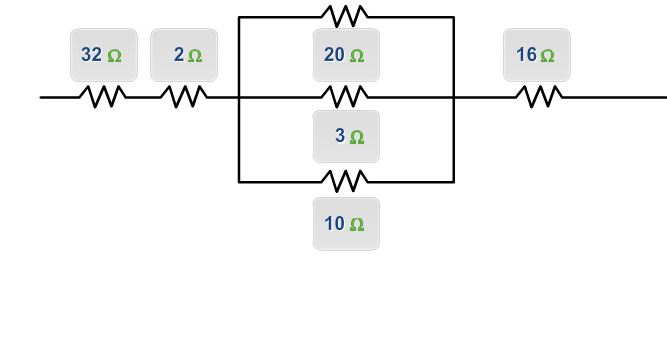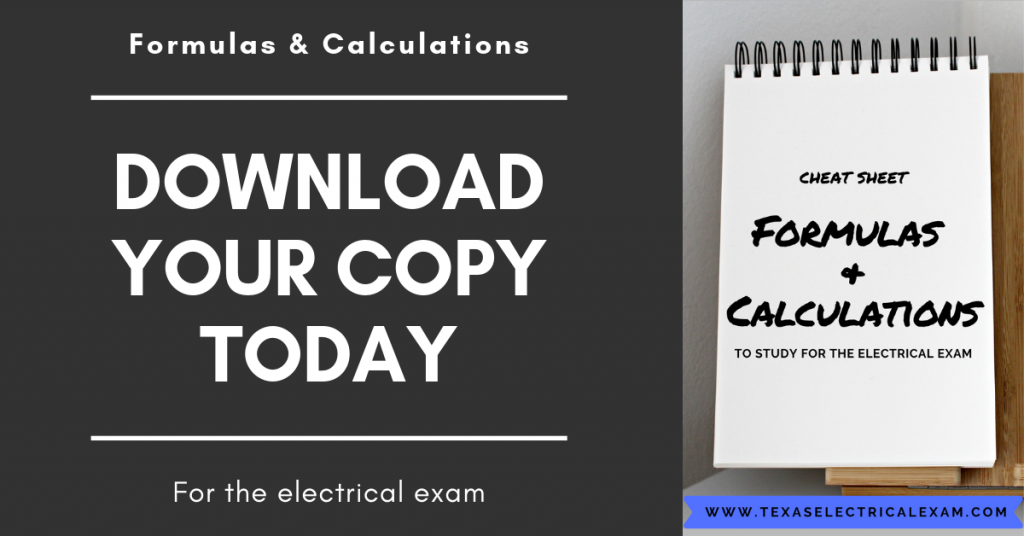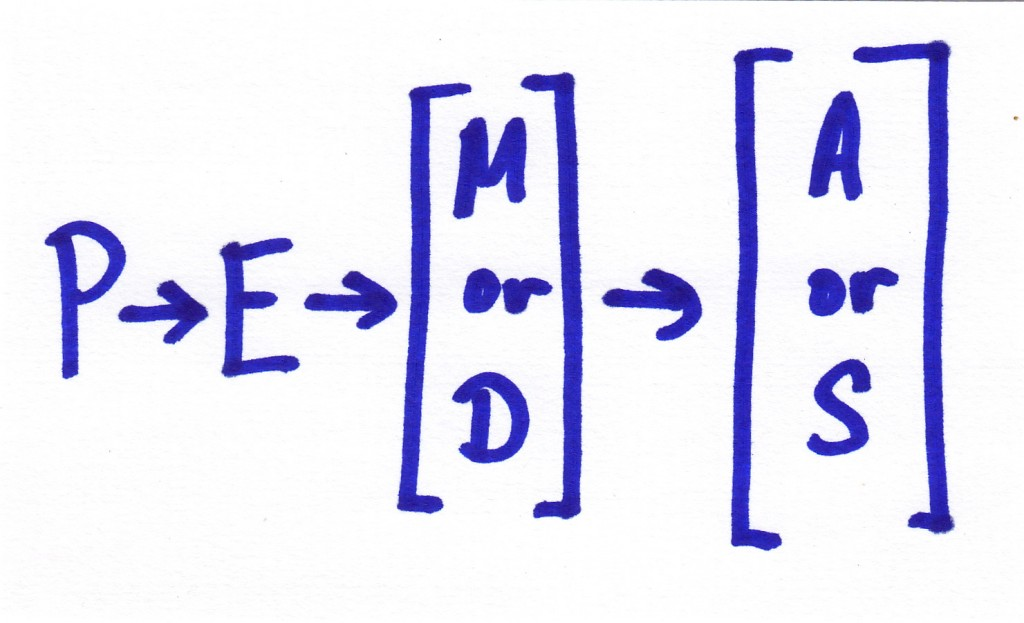# Must Have Formulas for Your Electrical Exam

Write these formulas for your electrical exam in your code book, you’ll be glad you did. Most testing centers allow short notes - only write important ones!

Before we go any further, click here to receive our Electrical Formulas Cheat Sheet.

Includes motor calculation steps, box fill, ranges, transformers, conduit fill, PIE, EIR, and more!The following images are from Mike Holt’s Illustrated Guide To Electrical Exam Preparation 2011 Edition and you can learn more about these formulas for your electrical exam here.  Questions in this book will help you understand then practice using these formulas.

Those of you preparing for the electrical exam based on the 2014 NEC should prepare with the 2014 Edition of the practice book.

##### UPDATE: As of this update 2020 will start rolling out soon. Check with your local municipal or state licensing department to see what edition you need to be studying for.  The formulas for your electrical exam should remain the same but always make sure.

In the test, you'll see questions that will ask you to determine the current of a circuit.  Also, the test will ask you to determine conductor resistance or even voltage drop (and more).  These formulas for your electrical exam are your core formulas. Memorize them but we also strongly suggest you write them in your book.  (Check with your examiner’s bulletin to make sure notes are allowed in your book).

In the off chance that notes are not allowed in your book, then make it a point to know these formulas in your sleep because you will need them.

## Most Common Formulas for Your Electrical Exam

#### Examples with formulas for your electrical exam

Question: What’s the resistance of the circuit conductors when the conductor voltage drop is 3V and the current flowing in the circuit is 100A?

(a) 0.03 ohms (b) 2 ohms (c) 30 ohms (d) 300 ohms

Answer: (a) 0.03 ohms

Step 1: What’s the question? What’s “R?”

Step 2: What do you know about the conductors? E = 3V dropped, I = 100A

Step 3: The formula is R = E/I

Step 4: The answer is R = 3V/100A

Step 5: The answer is R = 0.03 ohms

• • • • • •

Question: What’s the current flow in amperes through a 7.50 kW heat strip rated 230V when connected to a 230V power supply?

(a) 25A (b) 33A (c) 39A (d) 230A

Step 1: What’s the question? What’s “I?”

Step 2: What do you know? P = 7,500W, E = 230V.

Step 3: The formula is I = P/E.

Step 4: The answer is I = 7,500W/230V.

Step 5: The answer is 32.60A.

## Formulas for Your Electrical Exam - PEMDAS

Finally, technically this isn’t a formula but remember PEMDAS.  I can’t tell you how many students in our electrical exam prep seminars come to class and forget even the most basic order of operations.  Make it funny. Make it memorable. For the love of all you hold dear don’t miss questions for something so basic and elementary.

“Please Excuse My Drunk Aunt Sally”ORWhatever sticks around